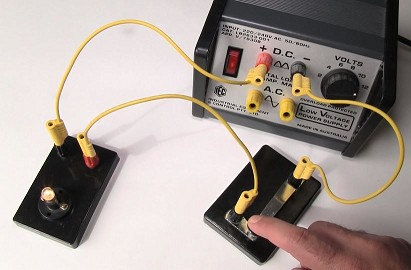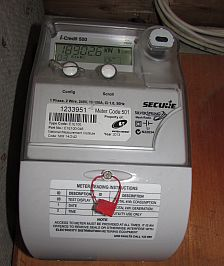Electric CircuitsAn Introduction to Series and Parallel Circuits. In this practical activity, students investigate series and parallel circuits. Beware of the short circuits though!

Designing Electric Circuits (pdf)  In this practical activity, which requires plenty of higher-order thinking, students have to design, construct, and draw up various electrical circuits according to set design briefs.
By signing each circuit as it is completed, teachers can ensure that the students have actually created the circuit and that they haven’t just copied from another group!

The Electric Circuits Extension Activities (pdf) is similar to the prac sheet above but the circuits require two-way switches.

It is assumed that the students have already been introduced to circuit symbols.The following activities are all about electricity usage in the home.

Electricity Usage. In this task students are asked to find out how much electricity they use over a period of a few days and to calculate the cost of that electricity. Some practice tasks are included on Page 2. For instructions on how to read an older-style meter (the ones that have the dials), click this link to access Ausgrid’s explanation. The newer “Smart Meters” are easy to read. Simply push the “Scroll” button to cycle through the display until you get to the display that says “kW h”. The meter in the picture says 18902.6 kW h. This is the total amount of kilowatt-hours that have been used since the meter was installed.

The Kilowatt-Hour. This worksheet allows students to calculate the energy use of a range of common appliances and the cost involved.